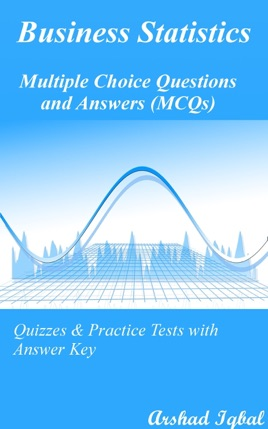• \$4.99

## Publisher Description

"Business Statistics Multiple Choice Questions and Answers (MCQs): Quizzes & Practice Tests with Answer Key" provides mock tests for competitive exams to solve 576 MCQs. "Business Statistics MCQ" PDF to download helps with theoretical & conceptual study for self-assessment, career tests. Business Statistics Quizzes, a quick study guide can help to learn and practice questions for placement test preparation.

Business Statistics Multiple Choice Questions and Answers PDF to download is a revision guide with a collection of trivia quiz questions and answers PDF on topics: Confidence intervals and estimation, data classification, tabulation and presentation, introduction to probability, introduction to statistics, measures of central tendency, measures of dispersion, probability distributions, sampling distributions, skewness, kurtosis and moments to enhance teaching and learning.

Confidence Intervals and Estimation MCQs: 21 Multiple Choice Questions. Data Classification, Tabulation and Presentation MCQs: 65 Multiple Choice Questions. Introduction to Probability MCQs: 64 Multiple Choice Questions. Introduction to Statistics MCQs: 64 Multiple Choice Questions. Measures of Central Tendency MCQs: 71 Multiple Choice Questions. Measures of Dispersion MCQs: 97 Multiple Choice Questions. Probability Distributions MCQs: 83 Multiple Choice Questions. Sampling Distributions MCQs: 53 Multiple Choice Questions. Skewness, Kurtosis and Moments MCQs: 58 Multiple Choice Questions.

Confidence Intervals and Estimation MCQs PDF: It covers quiz questions about introduction of estimation, confidence interval estimation, and sample statistics.

Data Classification, Tabulation and Presentation MCQs PDF: It covers quiz questions about data classification, data tables, data types, class width, frequency curve, frequency distribution types, and histograms.

Introduction to Probability MCQs PDF: It covers quiz questions about definition of probability, multiplication rules of probability, probability and counting rules, probability experiments, probability rules, Bayes theorem, relative frequency, rules of probability and algebra, sample space, and types of events.

Introduction to Statistics MCQs PDF: It covers quiz questions about introduction to statistics, data measurement in statistics, data types, principles of measurement, sources of data, statistical analysis methods, statistical data analysis, statistical techniques, structured data, and types of statistical methods.

Measures of Central Tendency MCQs PDF: It covers quiz questions about central tendency measures, arithmetic mean, averages of position, class width, comparison, measures of central tendency, harmonic mean, measurements, normal distribution, percentiles, relationship, median, mode, and mean.

Measures of Dispersion MCQs PDF: It covers quiz questions about measuring dispersion, arithmetic mean, average deviation measures, Chebyshev theorem, classification, measures of dispersion, distance measures, empirical values, interquartile deviation, interquartile range of deviation, mean absolute deviation, measures of deviation, squared deviation, standard deviation, statistics formulas, variance, and standard deviation.

Probability Distributions MCQs PDF: It covers quiz questions about binomial probability distribution, continuous probability distribution, discrete probability distributions, binomial distribution, expected value and variance, exponential distribution, hyper geometric distribution, normal distribution, Poisson distribution, random variable classes, rectangular distribution, standard normal probability distribution, statistics formulas, and uniform distribution.